# Addition of poles and zeros to the forward path transfer function

## Effect of addition of pole and zero to forward path transfer function

### Aim:

Study and plot unit step response of addition of pole and zero to the forward path transfer function for a unity feedback system. Plot the response for four different values of poles and zeros. Comment on the effect of addition of poles and zeros to forward path transfer function of a system.

### Theory:

Addition of pole to forward path transfer function:

The forward path transfer function of general second order system is given by,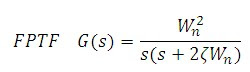Addition of pole to forward path transfer function:

When we add a pole, the transfer function becomes,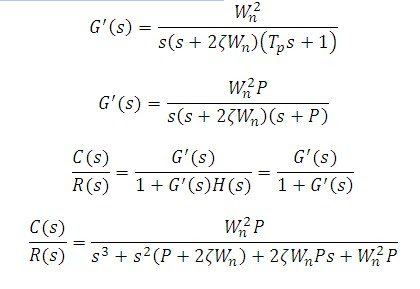MATLAB PROGRAM: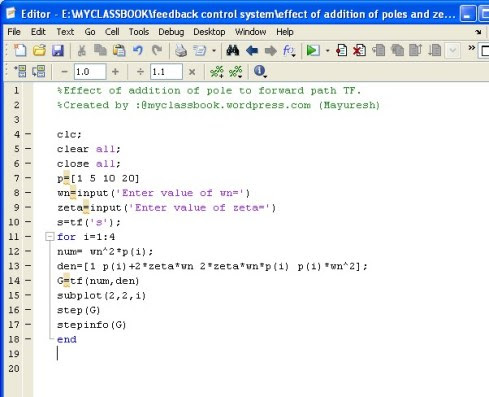addition of pole to forward path transfer function 1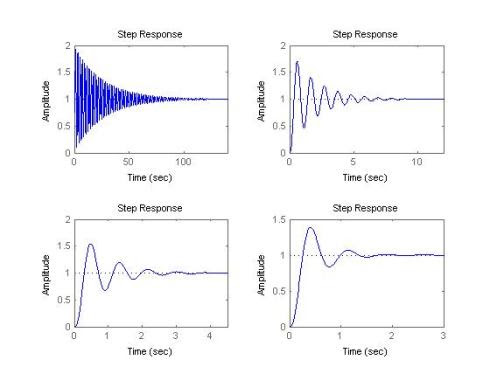addition of pole to forward path transfer function 1

Conclusion:

Effect of addition of pole to forward path transfer function:

1)      Unit step response of closed loop system when Wn=10, zeta=0.5, P=[1,5,10,20]. These responses show that addition of pole to forward path transfer function generally has the effect of increasing maximum overshoot of closed loop system.

2)      As the pole moves closer to the origin in s plane, the maximum overshoot increases.

3)      These responses also show that added pole increases rise time of step response.

Addition of zero to forward path transfer function:

The forward path transfer function of general second order system is given by,Addition of zero to forward path transfer function:

When we add a zero the forward path transfer function becomes,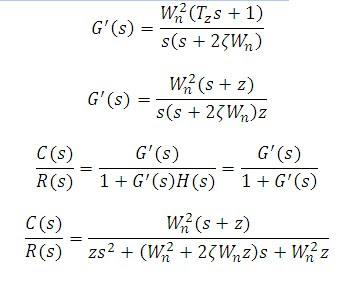MATLAB PROGRAM: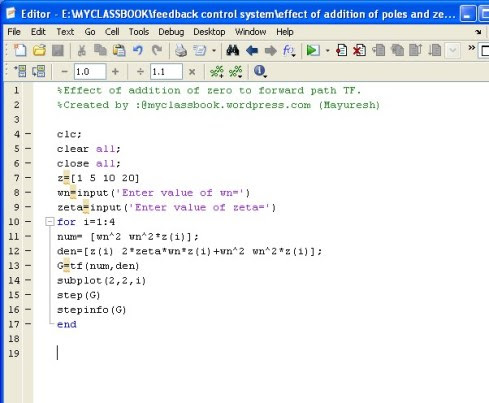addition of zero to forward path transfer function 1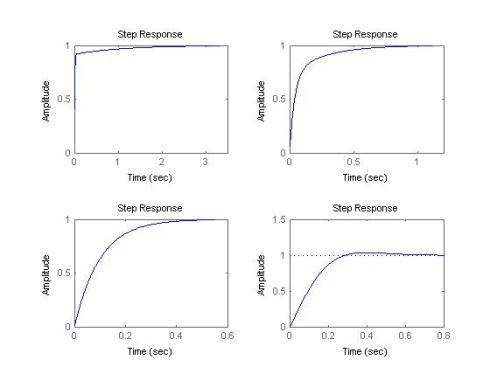addition of zero to forward path transfer function 1

Conclusion:

Effect of addition of zero to forward path transfer function:

1)      Addition of forward path zeros to left hand side of s plane increases speed of output response but a large overshoot may result if the zero is sufficiently lose to 0.

2)      The order of system does not increase with addition of zero to forward path transfer function.

3)      The zero in right half of s plane retards the system and produces an overshoot. The percentage overshoot decreases as the zero moves along positive real axis towards infinity. Again system oscillates for a large time.